# Emergent Behaviour, Population-based Search and Low-pass Filtering

Riccardo Poli, Alden Wright, Nic McPhee W. B. Langdon
Computer SciencePaper presented at CEC-2006 Pages 395-402

# Introduction

• Paper covers emergent behaviour in population based search (e.g. PSO and GAs). In some ways we can treat a group as like an individual.
• Mathematically model populations as a hill climber on equivalent smoother landscape (Using genetic program to find equivalent landscape).
• Schema theorem based proof that crossover smoothes landscapes
• Runs on rugged one-mix problem
• Conclusions

# Frequency Response for GA

• Present proof for two bits (large pop)
• But result can be generalised for any length bit string, any direction, any (homologous) crossover, at any rate, and with selection.
• Started Walsh analysis to give general frequency response.

# Crossover Smoothes Landscape

• Ignore drift by assuming large population.
• n(00) copies of string 00. Two bits, so pop is split between n(00) n(01), n(10) and n(11).
• The average slope in direction x2 is ½|n(11)-n(10)|+½|n(01)-n(00)|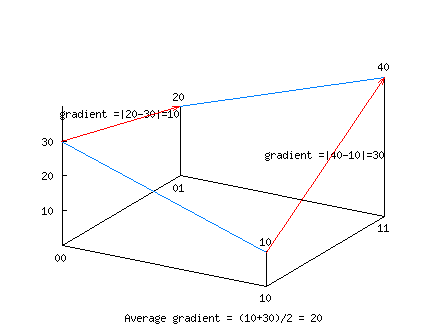# Use Schema Theorem

• 100% one point crossover exact schema theorem says n’(i,j)=n(i,*)n(*,j)
• Where n’ indicates next generation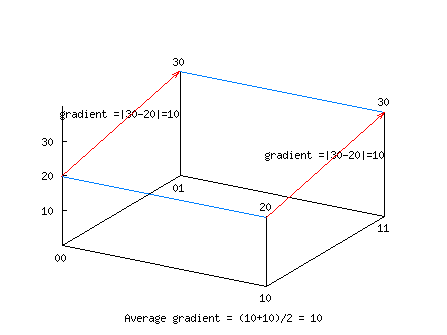# Average Slope in next generation

= ½|n’(11)-n’(10)|+½|n’(01)-n’(00)|
= ½|n(1*)n(*1)-n(*1)n(*0)|+½|n(0*)n(*1)-n(0*)n(*0)|
= ½n(1*)|n(*1)-n(*0)|+½n(0*)|n(*1)-n(*0)|
= ½(n(1*)+n(0*))|n(*1)-n(*0)|
= ½|n(*1)-n(*0)|
= ½|n(11)+n(01)- n(10)-n(00)|
= ½|n(11)-n(10) +n(01)- n(00)|
≤ ½|n(11)-n(10)|+½|n(01)- n(00)|

# Steps in Prove

• Average slope in next generation
• Use exact schema theorem on previous gen
• Move common factors outside | |
• Collect in () and | |
• Use n(1*)+n(0*) = n(**) = 1
• Re-express schema in terms of strings
• Re-order
• Use |a+b| ≤ |a|+|b|

# Crossover reduces slope

• Prove shows average gradient parallel to second dimension is smaller than it was before crossover.
• |a+b|=|a|+|b| only if sign(a)=sign(b).
• I.e. average slope is same only if two slopes we are averaging are either both up or both down.
• With L bits average over 2L-1 slopes. Unlikely all 2L-1 vectors have the same sign.

# Generalisation

• Paper contains proof of extension to
• Any direction parallel to co-ordinates
• Any length bit string
• Any type of crossover
• Any crossover rate
• In fact can also extend to include selection
• Obviously mutation tends to decrease number of popular strings and increase less numerous ones. I.e. to reduce slope

# Demonstration: OneMix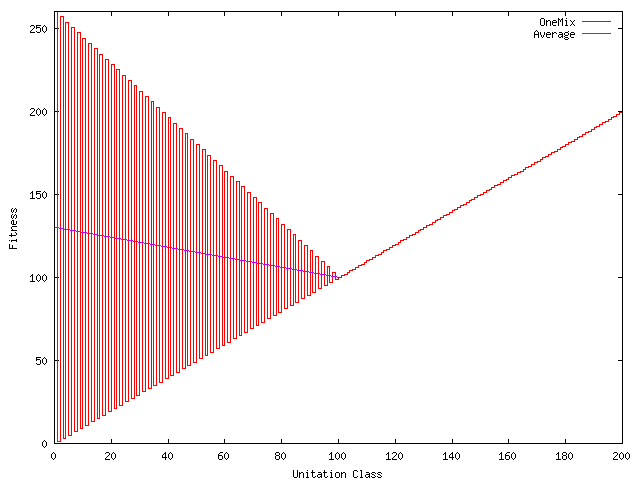# OneMix

• Half of OneMix is identical to OneMax
• But if less than half bits set, fitness varies rapidly with #bits set (i.e. unitation).
• Odd unitation values are still OneMax but if an even #bits set, OneMix like ZeroMax.
• Scaled so optimum is at 000...
• But average fitness near 000... is less than average near 111...

# GA and OneMix

• 1/L mutation and uniform crossover, binary tournaments, steady state pop=1000; GA filters landscape and population follows average to 111...
• GA with crossover responds more to low frequencies.
• 1/L mutation and no crossover, binary tournaments, steady state, pop=1000; population steps two bits at a time towards 000...
• GA with only mutation responds more to higher frequencies.
• By tuning balance between selection strength, mutation and crossover rates, the population can be driven to either extreme.

# Infinite Population Unitation Model

• Wright+Richter propose an infinite population model. It requires various assumptions but gives exact results with unitation problems of up to 60 bits.
• Plots on OneMix show:
• Selection tries to amplify high frequency components,
• Both mutation and crossover filter but crossover is more effective.

# OneMix Infinite Pop g=1 Crossover

Initial population is Gaussian.

Selection responds to all frequencies.

Crossover responds to low frequencies.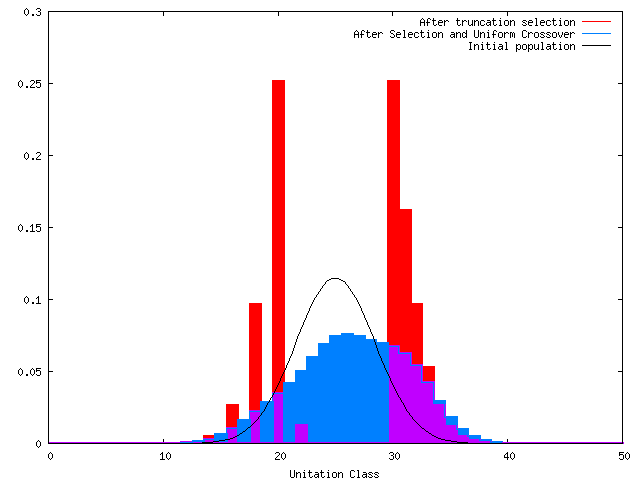# OneMix Infinite Pop g=1 Mutation

Selection responds to all frequencies.

Mutation responds to higher frequencies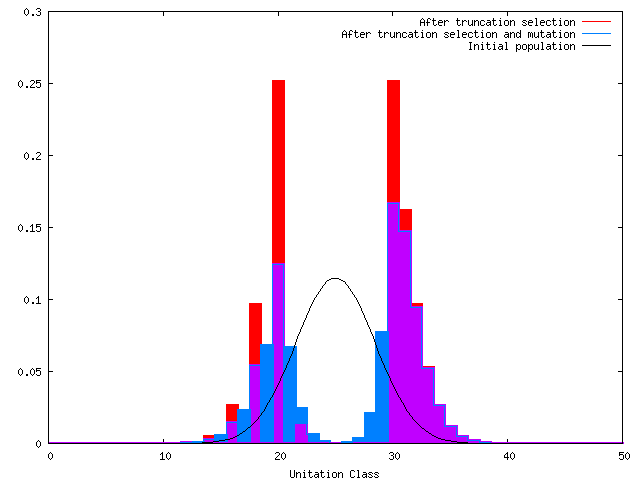# Conclusions

• In some ways a GA population behaves like a single individual moving on a transformed landscape.
• Population average moves like a hill climber on a filtered landscape. Can use genetic programming to find a suitable filter.
• Genetic operators (crossover and mutation) reduce gradient in the population, whilst selection tries to increase them.
• OneMix a useful test bed.

W.B.Langdon 25 July 2006